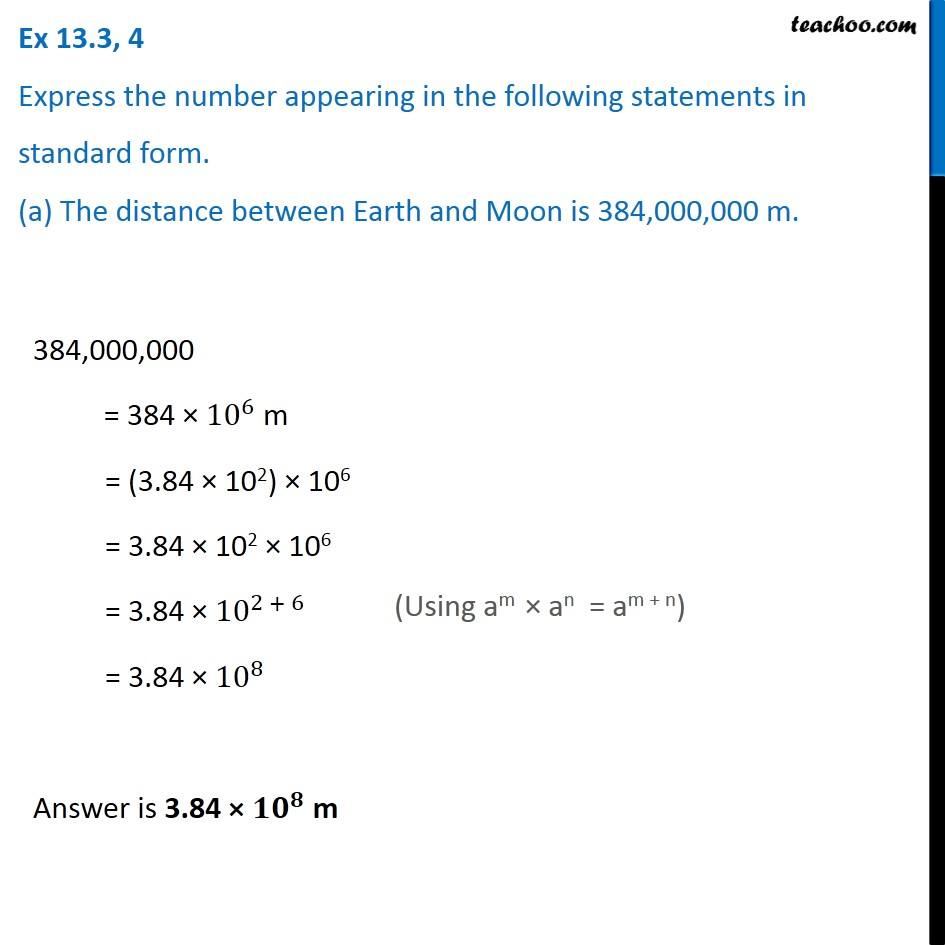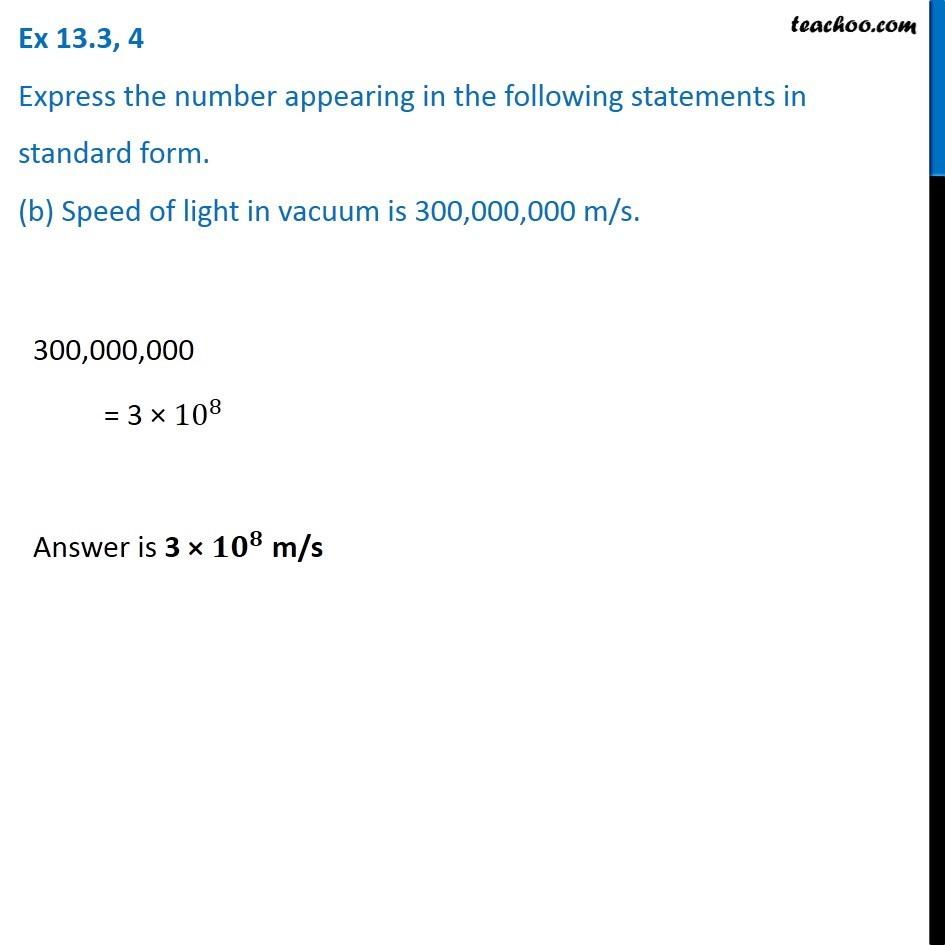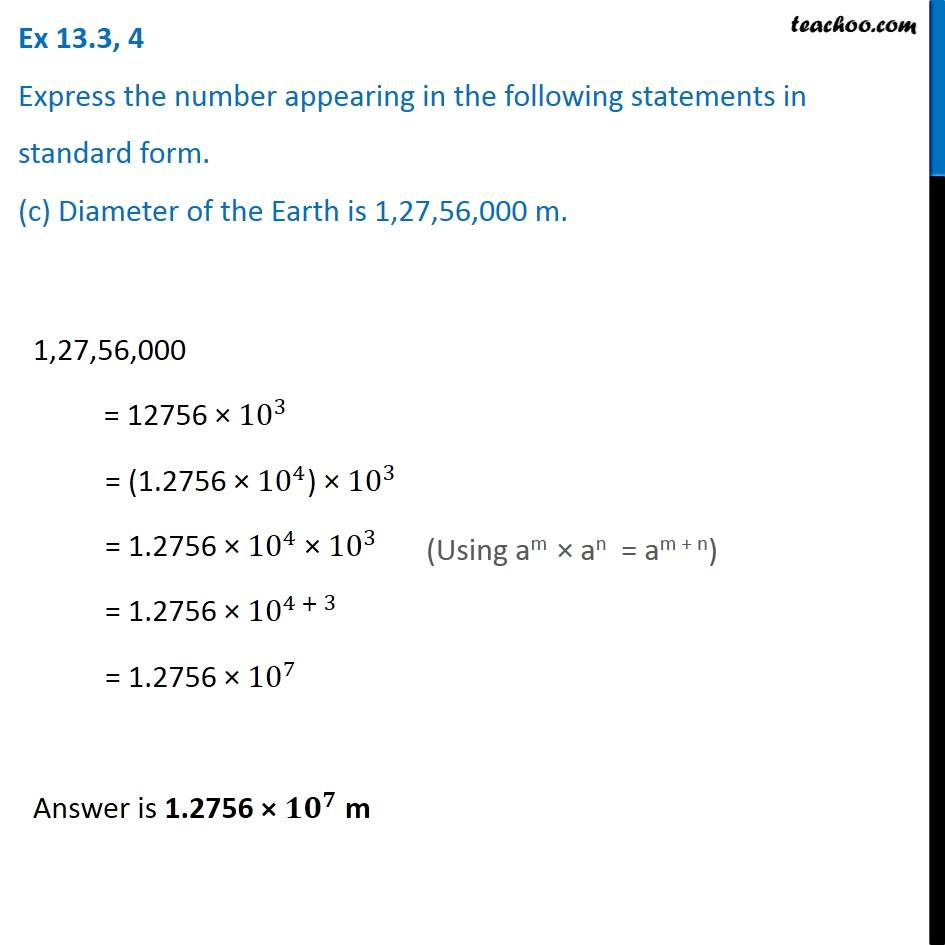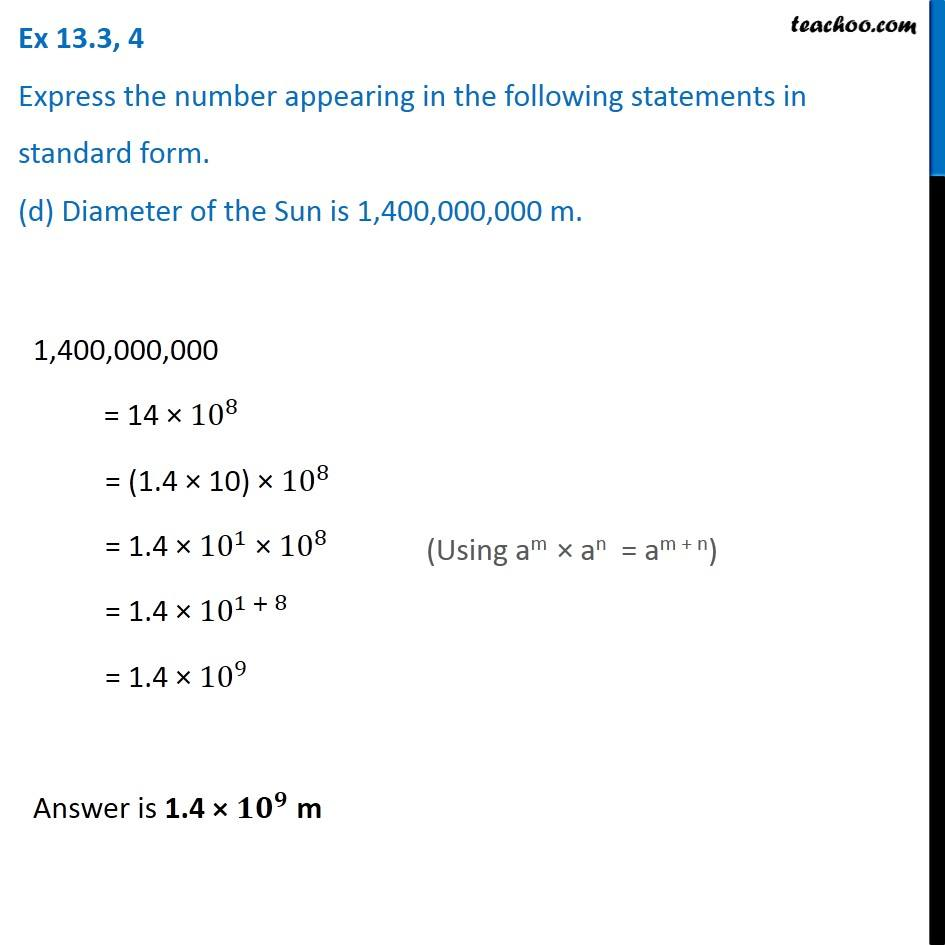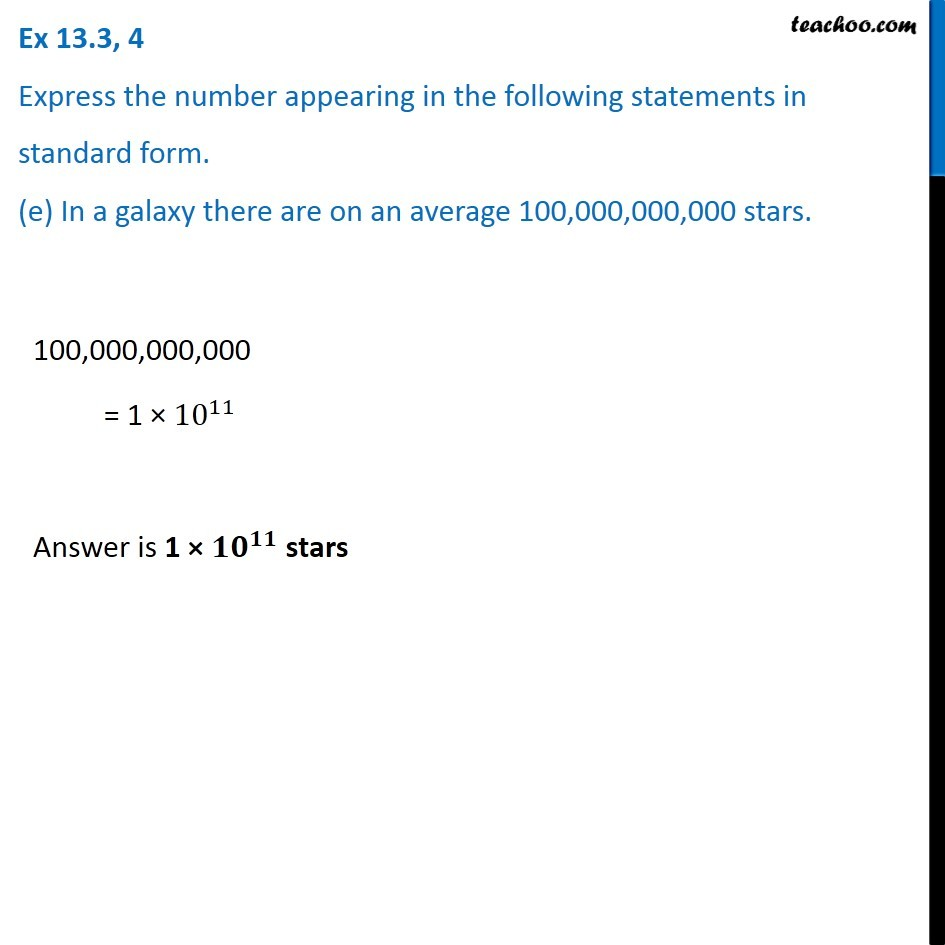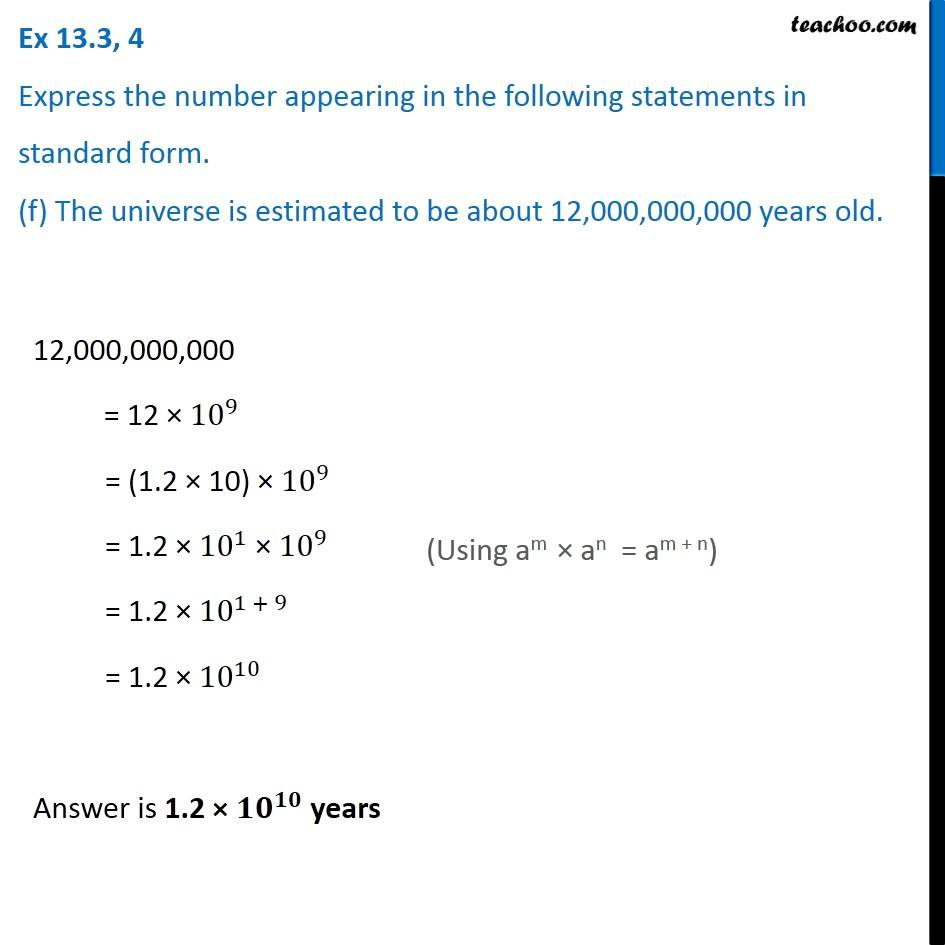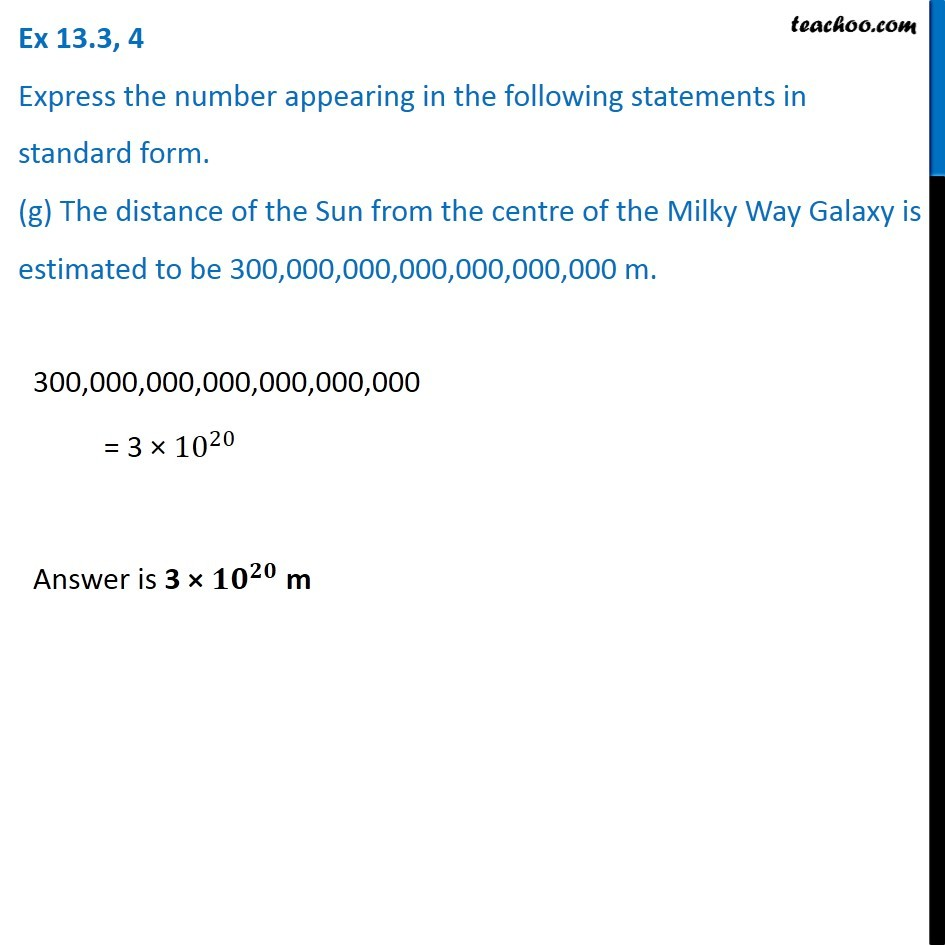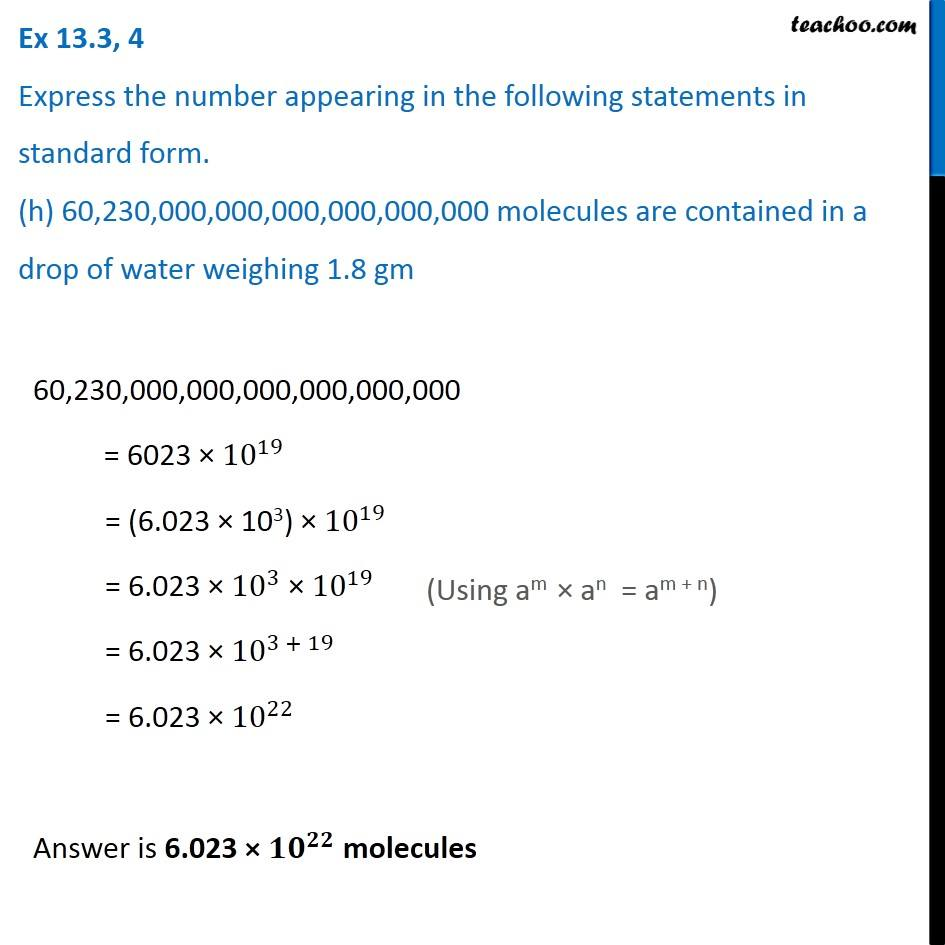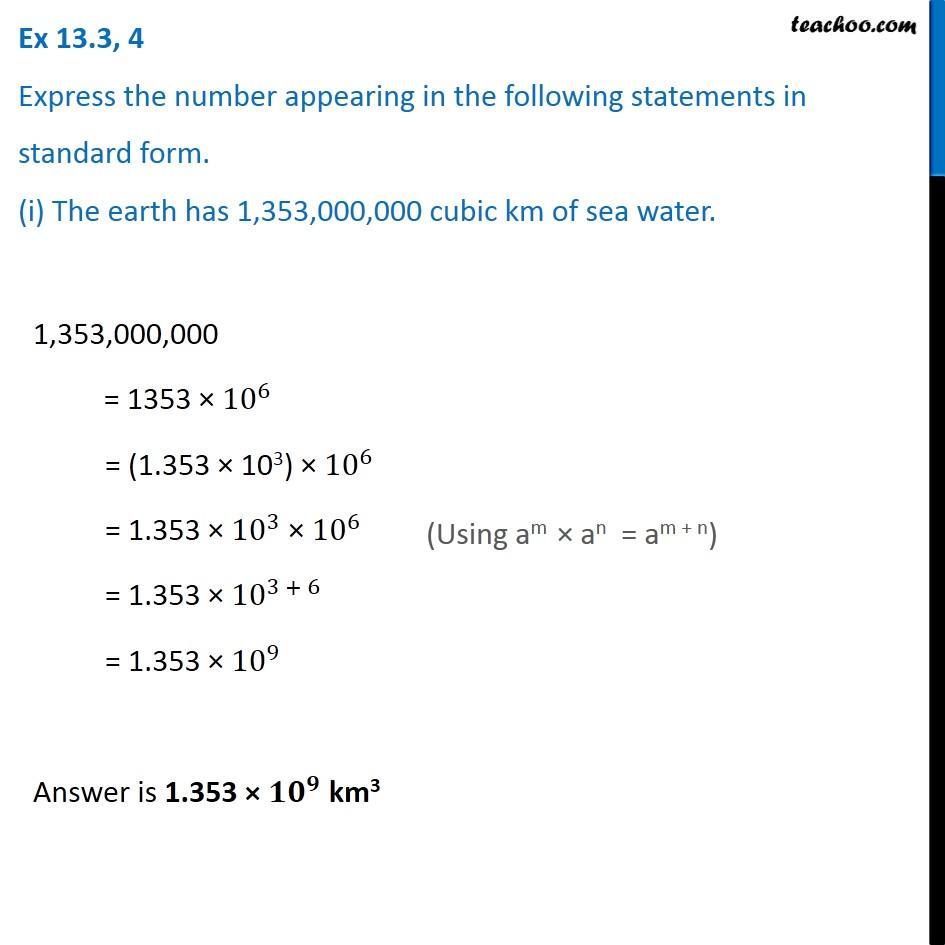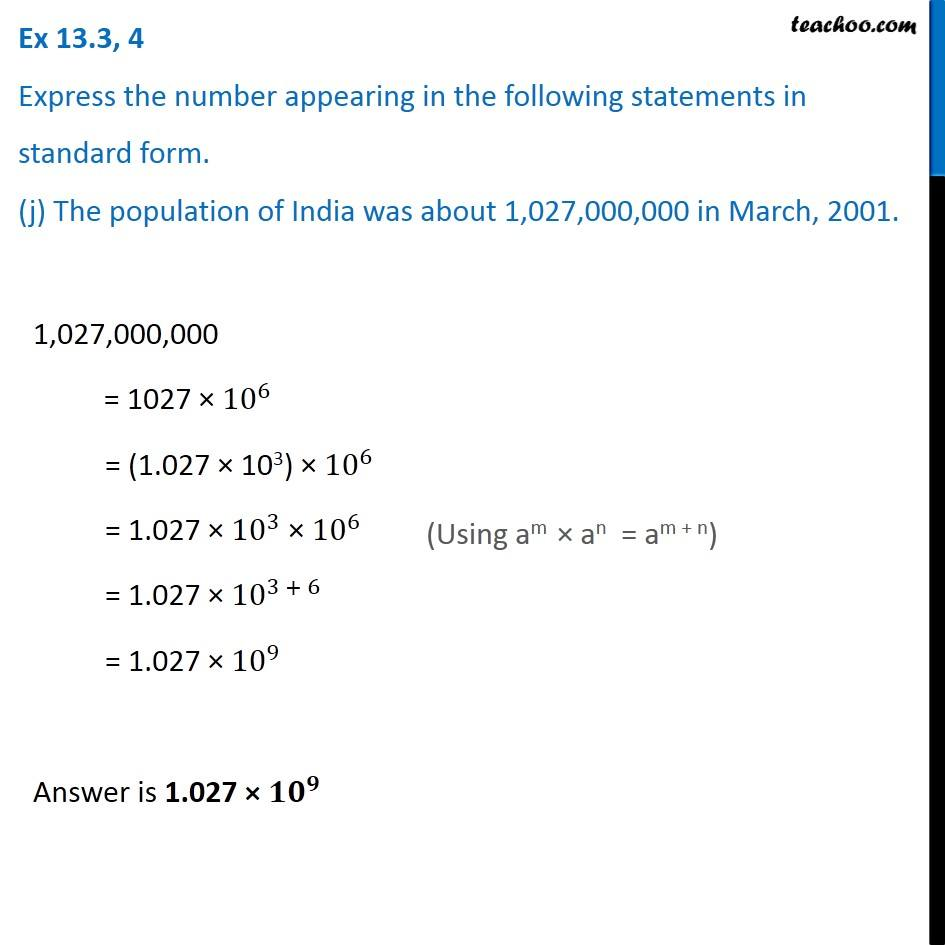Subscribe to our Youtube Channel - https://you.tube/teachoo

1. Chapter 13 Class 7 Exponents and Powers
2. Serial order wise
3. Ex 13.3

Transcript

Ex 13.3, 4 Express the number appearing in the following statements in standard form. (a) The distance between Earth and Moon is 384,000,000 m. 384,000,000 = 384 × 〖10〗^6 m = (3.84 × 102) × 106 = 3.84 × 102 × 106 = 3.84 × 〖10〗^(2 + 6) = 3.84 × 〖10〗^8 Ex 13.3, 4 Express the number appearing in the following statements in standard form. (b) Speed of light in vacuum is 300,000,000 m/s. 300,000,000 = 3 × 〖10〗^8 Answer is 3 × 〖𝟏𝟎〗^𝟖 m/s Ex 13.3, 4 Express the number appearing in the following statements in standard form. (c) Diameter of the Earth is 1,27,56,000 m. 1,27,56,000 = 12756 × 〖10〗^3 = (1.2756 × 〖10〗^4) × 〖10〗^3 = 1.2756 × 〖10〗^4 × 〖10〗^3 = 1.2756 × 〖10〗^(4 + 3) = 1.2756 × 〖10〗^7 Answer is 1.2756 × 〖𝟏𝟎〗^𝟕 m (Using am × an = am + n) Answer is 3.84 × 〖𝟏𝟎〗^𝟖 m (Using am × an = am + n) Ex 13.3, 4 Express the number appearing in the following statements in standard form. (d) Diameter of the Sun is 1,400,000,000 m. 1,400,000,000 = 14 × 〖10〗^8 = (1.4 × 10) × 〖10〗^8 = 1.4 × 〖10〗^1 × 〖10〗^8 = 1.4 × 〖10〗^(1 + 8) = 1.4 × 〖10〗^9 Answer is 1.4 × 〖𝟏𝟎〗^𝟗 m (Using am × an = am + n) Ex 13.3, 4 Express the number appearing in the following statements in standard form. (e) In a galaxy there are on an average 100,000,000,000 stars. 100,000,000,000 = 1 × 〖10〗^11 Answer is 1 × 〖𝟏𝟎〗^𝟏𝟏 stars Ex 13.3, 4 Express the number appearing in the following statements in standard form. (f) The universe is estimated to be about 12,000,000,000 years old. 12,000,000,000 = 12 × 〖10〗^9 = (1.2 × 10) × 〖10〗^9 = 1.2 × 〖10〗^1 × 〖10〗^9 = 1.2 × 〖10〗^(1 + 9) = 1.2 × 〖10〗^10 Answer is 1.2 × 〖𝟏𝟎〗^𝟏𝟎 years (Using am × an = am + n) Ex 13.3, 4 Express the number appearing in the following statements in standard form. (g) The distance of the Sun from the center of the Milky Way Galaxy is estimated to be 300,000,000,000,000,000,000 m. 300,000,000,000,000,000,000 = 3 × 〖10〗^20 Answer is 3 × 〖𝟏𝟎〗^𝟐𝟎 m Ex 13.3, 4 Express the number appearing in the following statements in standard form. (h) 60,230,000,000,000,000,000,000 molecules are contained in a drop of water weighing 1.8 gm 60,230,000,000,000,000,000,000 = 6023 × 〖10〗^19 = (6.023 × 103) × 〖10〗^19 = 6.023 × 〖10〗^3 × 〖10〗^19 = 6.023 × 〖10〗^(3 + 19) = 6.023 × 〖10〗^22 Answer is 6.023 × 〖𝟏𝟎〗^𝟐𝟐 molecules (Using am × an = am + n)

Ex 13.3